Springer Nature is making SARS-CoV-2 and COVID-19 research free. View research | View latest news | Sign up for updates

# Production scheduling with uncertain supply: a new solution to the open pit mining problem

## Abstract

The annual production scheduling of open pit mines determines an optimal sequence for annually extracting the mineralized material from the ground. The objective of the optimization process is usually to maximize the total Net Present Value (NPV) of the operation. Production scheduling is typically a Mixed Integer Programming (MIP) type problem containing uncertainty in the geologic input data and economic parameters involved. Major uncertainty affecting optimization is uncertainty in the mineralized materials (resource) available in the ground which constitutes an uncertain supply for mine production scheduling.

A new optimization model is developed herein based on two-stage Stochastic Integer Programming (SIP) to integrate uncertain supply to optimization; past optimization methods assume certainty in the supply from the mineral resource. As input, the SIP model utilizes a set of multiple, stochastically simulated scenarios of the mineralized materials in the ground. This set of multiple, equally probable scenarios describes the uncertainty in the mineral resource available in the ground, and allows the proposed model to generate a single optimum production schedule.

The method is applied for optimizing the annual production scheduling at a gold mine in Australia and benchmarked against a traditional scheduling method using the traditional single “average type” assessment of the mineral resource in the ground. In the case study presented herein, the schedule generated using the proposed SIP model resulted in approximately 10% higher NPV than the schedule derived from the traditional approach.

## Introduction

The problem of production scheduling in open pit mining is determining the parts of a mineralized deposit to be mined annually in an optimum order of sequence so as to maximize the total discounted profit. Solving the annual production scheduling problem in mining optimally is critical, because it determines the annual cash flows that can add several hundred million up to several billion dollars in magnitude, and at the same time it is based on an uncertain supply of mineralized materials for the resource available in the ground. This uncertainty is acknowledged in the related technical literature to be the major reason for not meeting production expectations (Baker and Giacomo 1998) or being the by far most dominant factor in failing mining projects (Vallee 2000). Given its substantial impact on the financial outcome of mining operations, this paper focuses on dealing with the uncertainty in metal content within a mineral deposit being mined.

Mining is an excavation activity in the earth made for the purpose of removal and sale of economically valuable minerals or materials. An orebody model containing the deposit attributes such as grades, tonnage, density, mining cost, processing cost, expected economic value etc. are used to determine the final pit limits. The final pit limits in mining can be defined as the limits of the deposit up to which it is economically feasible to mine. Lerchs and Grossmann’s algorithm (LG) based on graph theory (Lerchs and Grossmann 1965) and Maxflow algorithm based on network flow concept (Johnson 1968) are the most commonly used methods in practice. Hochbaum and Chen (2000) provided a critical review and discussion of various methods in finding optimum ultimate pit limits. Gershon (1983) presented a Mixed Integer Linear Programming (MILP) model formulation that an actual mining operation is represented as a mathematical model to maximize overall NPV from the operation. The paper stated that the models for optimising production scheduling of open pit mines require too many binary variables and cannot be solved. There have been some publications of methods which aim to reduce the required number of binary variables in MIP formulations for production scheduling in mining. In underground mines, Topal (2003) developed a methodology that substantially reduced the number of required binary variables by defining earliest start and latest start periods of the blocks through an intelligent process for Kiruna iron ore mine located in Sweden. To reduce the variables in optimizing production scheduling in open pit mining, Ramazan (2007) developed the Fundamental Tree Algorithm (FTA) that efficiently aggregates the blocks in an optimal way based on purely linear programming without using any integer variable. There are also heuristic approaches to aggregate the mining blocks that are discussed in Ramazan (1996) and Whittle (1988).

An estimated grade or mineral content of a block within an orebody model, representing a mineral deposit, is generated as a weighted average of the surrounding samples while the actual grade is unknown; uncertainty is caused by the single estimated value of a block that, in addition, cannot represent the possible in-situ grade variations between sampled points. Alternatively, stochastic spatial simulation (Boucher and Dimitrakopoulos 2009; Scheidt and Caers 2009; Mustapha and Dimitrakopoulos 2010) is a geostatistical approach used to generate multiple orebody models that are equi-probable representations of the actual mineral deposit in the ground. However, there is not one production scheduling method that can use multiple orebody models as input to generate an optimum production schedule in the presence of supply uncertainty. Grieco and Dimitrakopoulos (2007) proposed a probabilistic MIP method to optimize annual production scheduling in underground mining. Some past efforts in open pit mining included the sequential use of stochastic orebody models in traditional optimization methods by Ravenscroft (1992) and Dowd (1997). Dimitrakopoulos and Ramazan (2004) developed a long-term probabilistic type production scheduling method and introduced the concept of Geologic Risk Discounting (GRD). However, sequential processes and the probabilistic approach are shown to be inefficient and cannot produce an optimal schedule in the presence of uncertainty. Similar are the limitations of recent experimental approaches (Dimitrakopoulos et al. 2007; Godoy and Dimitrakopoulos 2004). Golamnejad et al. (2006) propose a chance-constrained formulation to account for grade uncertainty; however, chance-constrained formulations make severe and unrealistic assumptions, such as a Gaussian distribution and independence of the metal content (grade values) of mining blocks. Boland et al. (2008) consider a multistage stochastic programming approach, which however also makes unrealistic mining assumptions to be applicable. Dimitrakopoulos (2011) provides a review of stochastic approaches used in mine scheduling. Ramazan and Dimitrakopoulos (2004b) introduced a stochastic programming optimization model in a conference which was an initial start-up of this fully developed model proposed in this paper. The model produced promising results, but it did not include the formulation for leaching, the objective function did not have the stockpiling parts and model constraints were not presented and discussed in detail except the generic grade blending constraint. The major drawback of the publication was the case study, which considered a small scale two-dimensional hypothetical data set, which doesn’t guarantee the applicability of the model to a real mining operation with larger data.

In addition to the uncertainty of mineralized materials a mineral deposit can supply over time, there are other uncertainties in open pit mining that also play a significant role in defining the overall net profit expected from operations. These uncertainties include largely the fluctuating market demand for raw materials and metals affecting commodity prices, and production costs such as mining, processing, administration and so on. Technical and operational uncertainties, such as the uncertainties on the geotechnical parameters, introduce additional risk sources. The focus herein is on the uncertainty in metal content and supply as a starting point and due to its substantial impact on mining operations, as discussed above. Integrating additional sources of uncertainty in the present formulation or others remains a longer term research objective.

This paper proposes a new Stochastic Integer Programming model (SIP) that can optimize the annual production scheduling problem for open pit mines, considering uncertainty in the supply of mineralized materials extracted from the deposit. The proposed model is a novel approach in the sense that it can use multiple simulated orebody models without averaging the grade and contains parametric tools to manage the risk distribution effectively. The case study in a gold deposit in Australia shows that the SIP method has potential to improve the total NPV of mining projects substantially and it can be applied to large size open pit mines efficiently in terms of solution time of the large SIP model. In the following sections, the calculation of economic values is first discussed. The stochastic integer programming description and model formulation is presented after that. Subsequently, managing risk profile with the proposed method is discussed. Performance of the SIP model is analyzed and compared with a commonly used production scheduling method.

## Economic values of mining blocks

After an orebody model with geological and economic attributes is generated, the expected economic value of a block i E{V i } is calculated based on the expected Net Revenue (NR i ) to be gained from selling the contained metal within block i. If PC i is the processing cost (for all processes if multiple processing methods are used), the value of the block is:where MC i is the cost of mining, T i is the weight of the block; G i is the grade within block i; Rec is the processing recovery percentage. Note that in mining, a block is classified as “waste block” if processing cost is greater than the expected revenue from selling the contained metal within the block. It is classified as “ore block” if it is profitable to process and sell the contained metal.

## Stochastic integer programming for optimizing annual production scheduling in open pit mines

The proposed model offers a solution for the problem of which blocks should be mined in what time periods (years) without violating operational constraints (mining slope requirement, mining capacity, processing capacity, product qualities) so that the overall discounted profit from the sales of the products (metals) is maximized.

The following descriptions are adopted from Dimitrakopoulos and Ramazan (2009) where the generic two-stage recourse stochastic programming concept is explained specifically in mining terms. The two stage recourse model is a type of mathematical programming model where the fundamental mechanisms of anticipative and adaptive models are integrated within a single model. This model represents a trade-off between long term anticipatory strategies and the associated adaptive strategies. In mining terms, the trade-off could be between total expected NPV and associated risks in meeting production targets. Conceptually, a mathematical model is expected to result in higher NPV values if higher geological risks are tolerated within the model; a conservative risk approach is expected to result in a lower NPV value. The recourse problem can be expressed as follows:

Find $$x\in R^{n}_{+}$$, such that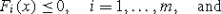(a1)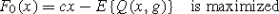(a2)

where

$$Q(x, g)=\inf \{q(g)y|W(x, g)=h(x, g)-T\};$$
(a3)

$$y\in R^{n'}_{+}$$; x is the matrix of decision variables $$(x_{i}^{t})$$ for deciding when to mine a block (if $$x_{i}^{t} =1$$, mine block i in period t). If all x variables are set to 0 or 1 values that represent percentage of blocks to be mined at each period t and if this set of x values are feasible for the model constraints under (a1), the values define a production schedule. Equation (a1) is a representation of all the constraints required for mining operations ((8), (9) and (10) in the subsequent section).

In (a2), cx is the total NPV value to be generated from the project given a decision on when the blocks should be mined, or given a production schedule defined by x-values. E{Q(x,g)} is the expected risk, or associated costs, of not meeting production targets under the chosen schedule. The risk in the model is defined as the deviations from the desired production targets and the unit cost multiplier matrix y as a function of the infeasibility. For a given schedule, x, and a set of grades, g, h(x,g) represents the tonne, grade and quality values to be produced periodically and T is the target matrix. Therefore, W(x,g) defines the risk and q(g)y defines the cost of the risk for the schedule, as a function of the uncertain grade values. After the true environment is observed through a simulated orebody model, the discrepancies that may exist between h(x,g) and T (for fixed x and observed h(x,g) and T) are calculated using (a3) as:

$$W(x, g) =h(x, g)-T$$

The cost of risk is defined in the objective function. The model takes the corrective or recursive form to re-define the x variables and the schedule so that NPV and cx are maximized, while the loss, q(g)y, is minimized. Therefore, an optimal decision x should modify the total expected profit to be generated by carrying out the plan, i.e. the direct NPV (cx) as well as the costs generated by the risk defined using simulated orebody models on the schedule, (E{Q(x,w)}).

The SIP model developed herein accounts for uncertain inputs for mineral supply in a deposit. This is achieved by simultaneously considering multiple, stochastically-simulated realizations of a deposit in the optimization process, which minimizes the risk of not meeting production targets caused by uncertain supply and, in addition, allows for the management of risk by controlling the risk profile of pertinent indicators. The model considers operational requirements, a main processor plant and also leaching as a possible alternative process. The stockpiling is also considered within the stochastic concept since the amount of material to be stockpiled will be affected by the block grades in the orebody model.

### Subscripts and superscripts

t :

is a scheduling time period;

g,o and q :

are target parameters, or type of production target; g is for the grade targets; o is for the ore production target; q is for the metal production target;

s :

is a simulated orebody model;

l :

is for the minimum target (lower bound);

u :

is for the maximum target (upper bound);

i :

is the block identifier.

### Variables to be determined

$$a_{\mathit{sl}}^{\mathit{tg}}, a_{\mathit{sl}}^{\mathit{to}}, a_{\mathit{sl}}^{\mathit{tq}},a_{\mathit{su}}^{\mathit{tg}}, a_{\mathit{su}}^{\mathit{to}}, a_{\mathit{su}}^{\mathit{tq}}$$ :

are the dummy variables (a-parameters) used to balance the equality constraints;

$$b_{i}^{t}$$ :

is a variable representing the fraction of block i mined in period t; if a $$b_{i}^{t}$$ variable is defined as binary (0, or 1), it is 1 if block i is mined in period t and 0 otherwise;

$$d_{\mathit{sl}}^{\mathit{tg}}, d_{\mathit{sl}}^{\mathit{to}}$$ and $$d_{\mathit{sl}}^{\mathit{tq}}$$ :

are the deficient amounts (d-parameters) for the target parameters produced below a desired minimum limit;

$$d_{\mathit{su}}^{\mathit{tg}}, d_{\mathit{su}}^{\mathit{to}}$$ and $$d_{\mathit{su}}^{\mathit{tq}}$$ :

are the excess amounts (d-parameters) for the target parameters produced above a desired maximum limit;

$$h_{s}^{t}$$ :

is the amount of material left in the stockpile at the end of period t with respect to orebody model s;

$$k_{s}^{t}$$ :

is the amount of material (ore) in tonnes processed from the stockpile with respect to orebody model s in period t;

$$w_{i}^{t}$$ :

is a variable representing the fraction of block i sent to the stockpile in period t.

### Known constants

$$C_{l}^{\mathit{tg}}, C_{l}^{\mathit{to}}, C_{l}^{\mathit{tq}},C_{u}^{\mathit{tg}}, C_{u}^{\mathit{to}}$$ and $$C_{u}^{\mathit{tq}}$$ :

are unit costs (C-parameters) for $$d_{\mathit{sl}}^{\mathit{tg}}$$, $$d_{\mathit{sl}}^{\mathit{to}},d_{\mathit{sl}}^{\mathit{tq}}, d_{\mathit{su}}^{\mathit{tg}}, d_{\mathit{su}}^{\mathit{to}}$$ and $$d_{\mathit{su}}^{\mathit{tq}}$$, respectively, in the objective function;

$$C_{l}^{t-} = C_{l}^{0-}/(1+ f_{l})^{t}$$; and $$C_{u}^{t-} = C_{u}^{0-}/(1+ f_{u})^{t}$$,:

where “−” after superscript t represents the type of targets g,o or q; f l and f u are the orebody risk discounting rates (uncertain mineral supply) used to calculate C-parameters;

$$E\{(\mathit{NPV})_{i}^{t}\}$$ :

is the expected (average) net present value (NPV) to be generated if block i is mined in period t. If the discount rate is r and $$E\{(\mathit{EV})_{i}^{0}\}$$ is the undiscounted expected economic value, it can be expressed as:

$$E\{(\mathit{NPV})_{i}^{t}\}= E\{(\mathit{EV})_{i}^{0}\}/(1+r)^{t}.$$
G tar :

is the targeted grade of the ore material to be processed;

G si :

is the grade of block i in orebody model s;

GST :

is the average grade of the stockpiled material;

GST min,GST max :

are minimum and maximum grades that a block is considered for stockpiling, respectively; the probability of a block to have a grade between GST min and GST max is the probability of that block to be considered for stockpiling;

h 0 :

is the amount of material already available in the stockpile;

M :

is the number of simulated orebody models;

$$\mathit{MC}_{i}^{t}$$ :

is mining cost of block i occurred in period t and discounted to time 0;

MCAP max :

is the total available mining capacity of the equipment;

MCAP min :

is the minimum amount of the material (waste and ore) required to be mined in each of the periods;

N :

is the number of blocks within final pit limits;

O tar :

is the targeted amounts of the ore material to be processed periodically;

O si :

is the ore tonnage within block i in orebody model s;

P :

is the total number of production periods, or mine life;

Q tar :

is the targeted amounts of the metal to be processed in a period;

Q si :

is the quantity of the metal in block i with respect to orebody model s;

(QST)t :

is the percentage of the metal content at the stockpile in period;

SV t :

is profit to be generated by processing a tonne of material from the stockpile in period t and discounted to time 0;

U :

is the number of blocks considered for stockpiling;

Y i :

number of blocks overlying ore block i considered for setting the slope constraints.

### Objective function

The objective function of the SIP model is constructed as the maximization of a profit function, defined as the difference between the total expected net present value and the cost of deviations from planned production targets. The objective function formulation is: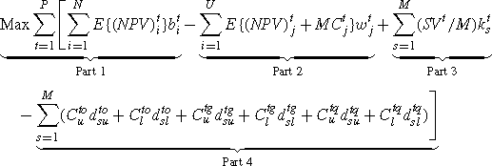(1)

The objective function in (1) has four parts: Part 1 refers to the expected total NPV to be generated if all the mined ore blocks were processed. Part 2 adjusts the economics of Part 1 for the percentage of the blocks that are sent to stockpile in a way that only mining costs are incurred in that time period. Part 3 adds the discounted profit to be generated by processing $$k_{s}^{t}$$ amount of material from the stockpile. Part 4 consists of geological risk management parameters.

It is fairly straightforward to calculate the economic values for a set of market parameters and set up the model in Part 1 and Part 2. In Part 3, the amount of material processed from the stockpile depends on the simulated orebody models. At first, the model makes the decision of when to mine a block without full information being known; i.e. the information about which simulated representation (model) of the orebody is the actual deposit. However, it assumes that the decision of how much material should be taken from the stockpile for processing can be made later in time when the mining occurs. At this time of mining complete information about the blocks is known: whether there is enough ore from the mine supplied to the processing plant or not and how much more material is needed for the plant. The unit value to be gained from processing material from stockpile is divided by M to account for the probability of a simulated orebody model to represent the actual deposit.

In cases where multiple independent processes such as concentrator and leaching are used, the proposed model should be implemented according to the situation of the operation. For example, if the leaching process is relatively small portion of the material processed, Part 4 of the equations should not be implemented for that process to minimize the dilution of the NPV with the parameters that their deviations are not expected to have significant impact on the overall NPV. For these secondary processes, we suggest implementing the grade and capacity constraints as a hard constraint as in (7a) and (7b) and using average orebody model. If each of the processes have significant impact on the revenue stream, then the full objective function and the related constraints (2), (3) and (4) should be implemented for each processing types.

The objective function of the proposed model contains multiple parameters with various coefficients. Gershon (1984) has noted that one must give extra care when assigning weights to multiple parameters in quantifying relative importance of them. Therefore, in the proposed SIP model, Part 4 is used to manage the uncertainty in the supply of ore from the deposit in the optimization process. The coefficients in front of these parameters define a risk profile for the production and NPV produced is the optimum for the defined risk profile. It is considered that if the expected deviations from the planned amount of ore tonnage having planned grade and quality in a schedule are high in actual mining operations, it is unlikely to achieve the resultant NPV of the planned schedule. Therefore, the SIP model contains the minimization of the deviations together with the NPV maximization to generate practical and feasible schedules and achievable cash flows. Section 4 provides further discussions on how these parameters can be used to control the distribution of risks over the time periods.

### Constraints

The deviation parameters in the objective function are calculated within the SIP model by the grade blending and processing plant constraints that consider each of the simulated orebody models.

### Grade blending constraints for each time period t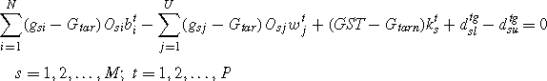(2)

### Processing constraints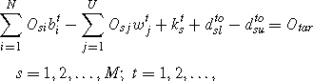(3)

### Metal production constraints for each time period t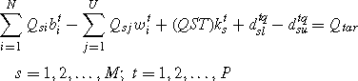(4)

### Stochastic stockpile constraints

Determine the quantity of material at the stockpile at the end of first period:(5a)

Determine the quantity of material at the stockpile at the end of period t (t>1):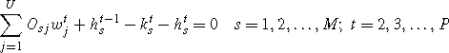(5b)

Capacity of the stockpile may be limited for each time period t: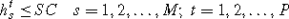(5c)

The amount of material that can be taken from the stockpile cannot be more than the available amount at the stockpile at the end of previous period: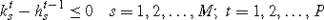(5d)

A block must be mined before stockpiling.(6)

To increase the efficiency of the SIP model, the optimization is applied in two steps for large size problems. Initially, the model is formulated without including the main stochastic constraints (2), (3) and (4) that are feasible for any value of the binary $$(b_{i}^{t})$$ variables. Instead of these main stochastic constraints, the following mill input capacity and metal production constraints are first applied:

### Processing capacity step 1 constraints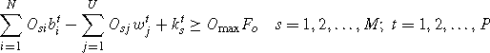(7a)

### Metal production step 1 constraints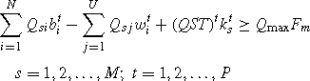(7b)

where, F o and F m are fractions for ore and metal production to limit the initial results; min and max are some values around the targeted value. Presumably (7a) and (7b) hold for all s and t. For example, an initial schedule can be generated in such a way that F o , assumed as 70% of the processing capacity, must be reached with respect to each of the simulated orebody models. Initially, a high probability such as 90% is applied. This means the capacity constraints must hold for at least 90% of the simulated orebody models. If the result is infeasible, the probability can be decreased 5 or 10% at a time. The SIP model with these hard constraints is solved faster than the SIP model with the stochastic constraints. Then, the model is formulated again without (7a), (7b), but including (2), (3) and (4). The solution generated from the initial model is used as a starting solution for the SIP model. This procedure often significantly reduces the solution time for the large SIP model.

### Slope constraints

Slope constraints ensures that to mine a specific block, all the blocks overlying that specific block must be mined.

### For each overlying block of the block i and each period t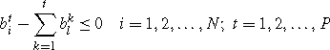(8)

### Reserve constraints

A block has to be mined fully (100%)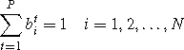(9)

### Mining capacity constraints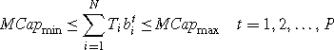(10)

### Binary definition

In mining, a block is usually mined completely within a period of a year during the scheduling process. To mine the entire block, the block variables are traditionally defined as binary; 1 if the block is mined in a period and 0 if not mined. However, this logic is difficult to implement in real mining cases due to large size of block models that MIP formulations cannot solve. Therefore, only the variables representing ore blocks are defined as binary and the other variables are continuous (linear). Since waste blocks are linked to ore block mining as discussed in Ramazan and Dimitrakopoulos (2004a), waste block variables would be forced to produce binary results.

## Managing risk profiles using the SIP model

The risk management process in the presence of uncertain mineral supply when using the proposed SIP model is demonstrated in this section using a relatively small pushback, containing 1064 blocks, from a gold deposit that can be mined within 3 years. To protect data confidentiality and without loss of generality, the production rates are given as percentages and an explanation of using the SIP model to control the risk profile is given. The probability and distribution of the geological risk can be managed between scheduling periods. More specifically, Fig. 1 shows deviations from metal production between scheduling periods for a given schedule. The aim was to mine the least risky part of the deposit early in the mine life and delay the mining of high uncertainty regions for later periods. This is done assuming that more information would become available in time and future planning would be more confident. It is very important in mining operations to meet the production targets at early years of the mine life so that the capital spent can be recovered earlier. For this reason, the geological risk discounting (GRD) is used in the SIP model. The first year production is very likely to meet the production targets. During the second year, the chance of shortage in metal production is higher than the first year, because the cost of deviating from the target in the second year is less than the cost for the first year in the objective function. The deviations are very high in the last year because of depletion of the deposit. However, if the deposit contained sufficient metal, the chance of higher deviations would still be expected as compared to the previous periods, because the cost of deviations in the last year was less than the previous years. Theoretically, the rate of variations in the magnitude of the deviations and chances of the deviations would increase as the GRD factor applied increases and would decrease as the GRD rate decreased. If a balanced-risk profile is to be produced, GRD must be set to 0.

It should be noted that there is no control of the distribution of risk profile in traditional production scheduling methods since they can only use a single orebody model as input. The risk profile on a schedule by traditional methods is random. However, one needs to be aware of the fact that the SIP model cannot control the risk distribution 100%, although it can provide substantial control as illustrated in Fig. 1. The risk distribution is also dependent on how the grade variability is spread throughout the mineralized region. Some blocks may have to be mined due to slope constraints or other operational reasons at earlier periods even if they contain high uncertainty in the estimated grades. This type of grade uncertainty can only be reduced through drilling and sampling process in the high risk areas that need to be mined during earlier periods.

In this paper, it is suggested that a series of schedules be produced with different C-parameters, which may include actual approximated cost numbers where possible based on some reasonable assumptions, and GRD factors. The resultant NPV values and risk profiles need to be analyzed using simulated orebody models and decisions must be based on the analysis considering the company’s objectives.

## Conclusions

In this paper, a new stochastic integer programming model is developed and applied to a gold deposit in Australia. The proposed SIP model uses multiple conditionally simulated orebody models that are equi-probable representations of the actual deposit in the ground for optimizing annual production schedules in open pit mines. This approach accounts for the uncertainty in the mineral supply from the deposit in the ground, unlike the traditional scheduling methods that are based on a single orebody model assumed to be the actual deposit in the ground being mined.

Incorporating multiple conditionally simulated orebody models without averaging the grades in a mathematical optimization model delivers a risk robust production schedule. The robustness of the schedule comes from the fact that the effect of the geological uncertainty (variability of metal content in the ground) on the periodical production targets can be managed using the model parameters.

The SIP model is found to be a powerful tool to manage the risk of not meeting production targets by controlling the magnitude and the probability of the risk within individual production periods and, in addition, controlling how the risk is distributed between production periods. The model allows the user to define the risk profile and generates the schedule with the optimum NPV for the resultant risk profile. The risk profile in the schedules that are generated by traditional methods is random and can lead to substantial losses, even premature closure of a mining operation, by failing to meet the planned production targets

The proposed SIP model is very efficient in that it needs no more binary variables than the traditional MIP formulations using single orebody model as input. The total number of binary variables representing the blocks for scheduling periods in the SIP formulation using many simulated orebody models is the same as in the MIP formulations using single orebody models. The only additional variables are the deviation variables and some variables to control the stockpile, which are defined as continuous. The number of constraints is larger in the SIP scheduling formulation than the traditional MIP formulations. However, possible inefficiency issues caused by this increased number of constraints can be managed as discussed in this paper.

In this study, by defining only ore block variables as binary and leaving the waste block variables as continuous, the SIP model could be applied efficiently to an Australian gold deposit and is shown to increase the overall NPV for about 10%. As well established in general terms (e.g. Birge and Louveaux 1997), the value generated using stochastic solutions is always greater than, or equal to, the value that can be obtained using a deterministic approach. In open pit mining applications, tests have shown that stochastic approaches produce a 10 to 25% of additional value for mining operations than the traditional scheduling methods.

## References

1. Baker CK, Giacomo SM (1998) Resource and reserves: their uses and abuses by the equity markets. In: Ore reserves and finance: a joint seminar between Australasian Institute of Mining and Metallurgy (AusIMM) and Australian Securities Exchange (ASX), Sydney

2. Birge JR, Louveaux F (1997) Introduction to stochastic programming. Springer series in operations research. Springer, Berlin

3. Boland N, Dumitrescu I, Froyland G (2008) A multistage stochastic programming approach to open pit mine production scheduling with uncertain geology. Optimization Online. http://www.optimization-online.org/DBHTML/2008/10/2123.html

4. Boucher A, Dimitrakopoulos R (2009) Block simulation of multiple correlated variables. Math Geosci 41:215–237

5. Dimitrakopoulos R (2011) Stochastic optimization for strategic mine planning: a decade of developments. J Min Sci 84, 138–150

6. Dimitrakopoulos R, Ramazan S (2004) Uncertainty based production scheduling in open pit mining. SME Trans 316:106–112

7. Dimitrakopoulos R, Ramazan S (2009) Stochastic integer programming for optimising long-term production schedules of open pit mines: methods, application and value of stochastic solutions. Trans Inst Min Metall Ser A, Min Ind 117(4):155–160

8. Dimitrakopoulos R, Martinez L, Ramazan S (2007) A maximum upside/minimum downside approach to the traditional optimization of open pit mine design. J Min Sci 43(1):81–90

9. Dowd PA (1997) Risk in minerals projects: analysis, perception and management. Trans Inst Min Metall Ser A, Min Ind 106:9–18

10. Gershon ME (1983) Optimal mine production scheduling evaluation of large scale mathematical programming approaches. Int J Min Eng 1:315–329

11. Gershon ME (1984) The role of weights and scales in the application of multiobjective decision making. Eur J Oper Res 15:244–250

12. Godoy M, Dimitrakopoulos R (2004) Managing risk and waste mining in long-term production scheduling. SME Trans 316:43–50

13. Golamnejad J, Osanloo M, Karimi B (2006) A chance-constrained programming approach for open pit long-term production scheduling in stochastic environments. J S Afr Inst Min Metall 106:105–114

14. Grieco NJ, Dimitrakopoulos R (2007) Managing grade risk in stope design optimisation: probabilistic mathematical programming model and application in sublevel stoping. Trans Inst Min Metall Ser A, Min Ind 116:49–57

15. Hochbaum DS, Chen A (2000) Performance analysis and best implementations of old and new algorithms for the open-pit mining problem. Oper Res 48:894–913

16. Johnson TB (1968) Optimum open pit mine production scheduling. PhD dissertation, University of California, Berkeley

17. Lerchs H, Grossmann IF (1965) Optimum design of open-pit mines. Trans CIM LXVII:47–54

18. Mustapha H, Dimitrakopoulos R (2010) High-order stochastic simulation of complex spatially distributed natural phenomena. Math Geosci 42:457–485

19. Ramazan S (1996) A new push back design algorithm in open pit mining. MSc thesis, Department of Mining Engineering, Colorado School of Mines, Golden, Colorado, USA

20. Ramazan S, Dimitrakopoulos R (2004a) Recent applications of operations research and efficient MIP formulations in open pit mining. SME Trans 316:73–78

21. Ramazan S, Dimitrakopoulos R (2004b) Stochastic optimisation of long-term production scheduling for open pit mines with a new integer programming formulation. In: Orebody modelling and strategic mine planning. Spectrum series, vol 14, pp 353–360. The Australian Institute of Mining and Metallurgy (AusIMM), Victoria

22. Ramazan S (2007) The new fundamental tree algorithm for production scheduling of open pit mines. Eur J Oper Res 177:1153–1166

23. Ravenscroft PJ (1992) Risk analysis for mine scheduling by conditional simulation. Trans Inst Min Metall Ser A, Min Ind 101:A104–A108

24. Scheidt C, Caers J (2009) Respresenting spatial uncertainty using distances and kernels. Math Geosci 41:397–419

25. Topal E (2003) Advanced underground mine scheduling using mixed integer programming. PhD thesis, Colorado School of Mines, Golden, CO

26. Vallee M (2000) Mineral resource + engineering, economic and legal feasibility = ore reserve. Can Min Metall Bull 93:53–61

27. Whittle J (1988) Beyond optimization in open pit design. In: Proc Canadian Conf on Computer Applications in the Mineral Industries, Rotterdam, pp 331–337

## Acknowledgements

The authors would like to acknowledge substantial financial contributions from AngloGold Ashanti, BHP Billiton, Rio Tinto and Xstrata Copper. The authors also acknowledge with thanks that the simulated orebody models used in this study were generated by Dr. Marcelo Godoy.

## Author information

Correspondence to Salih Ramazan.

## Rights and permissions

Open Access This is an open access article distributed under the terms of the Creative Commons Attribution Noncommercial License (https://creativecommons.org/licenses/by-nc/2.0), which permits any noncommercial use, distribution, and reproduction in any medium, provided the original author(s) and source are credited.

Reprints and Permissions

Ramazan, S., Dimitrakopoulos, R. Production scheduling with uncertain supply: a new solution to the open pit mining problem. Optim Eng 14, 361–380 (2013). https://doi.org/10.1007/s11081-012-9186-2